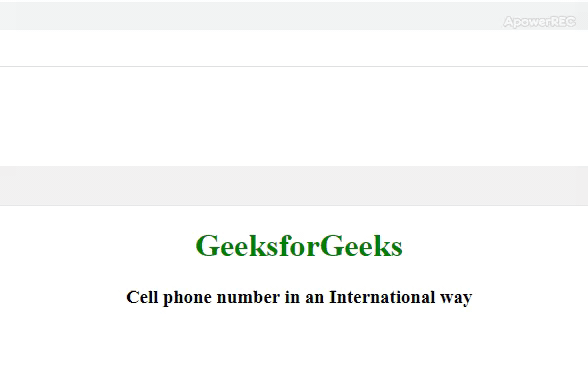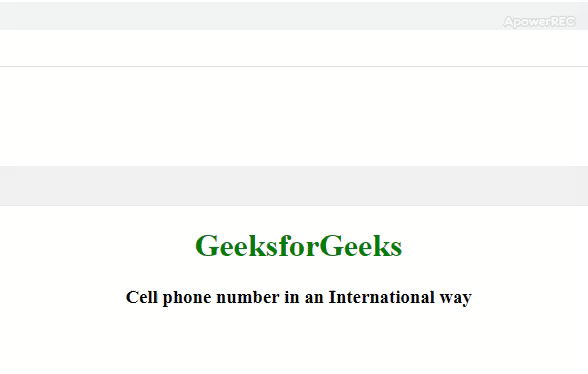Skip to content
Related Articles
How to write a cell phone number in an international way using JavaScript ?
• Last Updated : 31 Dec, 2019

E.164 format is used to convert a phone number into an international format. It is an internationally recognized standard that defines a general numbering plan.

The international number format according to E.164 is as follows:

`[+][country code][area code][local phone number]`
• +: Plus sign
• country code: International country code. It comes after the plus sign. For ex. It is +91 for India whereas it is +1 for USA.
• area code: It follows after the international country code. India area codes usually have 2, 3 or 4 digits. For ex. In India, Kolkata has an area code of 3211 and Mumbai has area code of 22.
• local phone number: Local phone number

Prerequisite article: How to write Regular Expressions?

Concept of regular expressions is used for converting a cell phone number into international way. Regular expressions are a generalized way to match patterns with sequences of characters.
Some examples according to the E.164 format

```Without international code
localNumber: 9760064000
intlNumber: (976) 006-4000

With international code
localNumber: 919760064000
intlNumber: +91 (976) 006-4000
```

Example 1: This code uses the regular expression /^(\d{3})(\d{3})(\d{4})\$/ for validating the phone numbers. If the number is found to be valid then an array will be returned and if not then null will be returned. The elements of the returned array is then joined according to the E.164 format for international number.

• Program:
 ```<``html` `lang``=``"en"``>`` ` `<``head``>``    ``<``meta` `charset``=``"UTF-8"``>``    ``<``meta` `name``=``"viewport"``        ``content``=``"width=device-width, initial-scale=1.0"``>``    ``<``meta` `http-equiv``=``"X-UA-Compatible"``        ``content``=``"ie=edge"``>``    ``<``title``>``        ``Cell phone number in an International way``    ````     ` `    ``<``style``>``        ``body {``            ``text-align: center;``        ``}``         ` `        ``h1 {``            ``color: green;``        ``}``    `````` ` `<``body``>``    ``<``h1``>GeeksforGeeks``     ` `    ``<``h3``>``        ``Cell phone number in``        ``an International way``    ````     ` `    ``<``script``>``        ``var localNumber = prompt("Please enter your number");`` ` `        ``// Using regular expression to check whether``        ``// string is valid or not``        ``var newArray = localNumber.match``                ``(/^(91|)?(\d{3})(\d{3})(\d{4})\$/);`` ` `        ``// Checking the international code``        ``var intlCountryCode = (newArray ? '+91' : '');`` ` `        ``// Resolving the above array we get``        ``// the international number``        ``var internationalNumber = intlCountryCode +``                ``' (' + newArray + ') ' + newArray``                ``+ '-' + newArray;`` ` `        ``document.write("The number in international" + ``                    ``"form is: " + internationalNumber);``    `````` ` ``
• Output:Example 2: This code uses the regular expression /^(91|)?(\d{3})(\d{3})(\d{4})\$/ for validating the phone numbers with international code. The same approach as above is followed to get the phone number in international format.

• Program:
 ```<``html` `lang``=``"en"``>``<``head``>``    ``<``meta` `charset``=``"UTF-8"``>``    ``<``meta` `name``=``"viewport"``        ``content``=``"width=device-width, initial-scale=1.0"``>``    ``<``meta` `http-equiv``=``"X-UA-Compatible"``        ``content``=``"ie=edge"``>``    ``<``title``>``        ``Cell phone number in an International way``    ````     ` `    ``<``style``>``        ``body {``            ``text-align: center;``        ``}``         ` `        ``h1 {``            ``color: green;``        ``}``    ``````<``body``>``    ``<``h1``>GeeksforGeeks``    ``<``h3``>``        ``Cell phone number in``        ``an International way``    ````     ` `    ``<``script``>``     ` `    ``var localNumber= prompt("Please enter your number");``     ` `    ``// Using regular expression to check``    ``// whether string is valid or not``    ``var newArray = localNumber.match``                ``(/^(91|)?(\d{3})(\d{3})(\d{4})\$/);``     ` `    ``// Checking the international code``    ``var intlCountryCode=(newArray?'+91':'');``     ` `    ``// Resolving the above array we get``    ``// the international number``    ``var internationalNumber = intlCountryCode + ' ('``                ``+ newArray + ') ' + newArray``                ``+ '-' + newArray;``     ` `    ``document.write("The international number is: "``                ``+ internationalNumber); ``    `````` ` ``
• Output:My Personal Notes arrow_drop_up